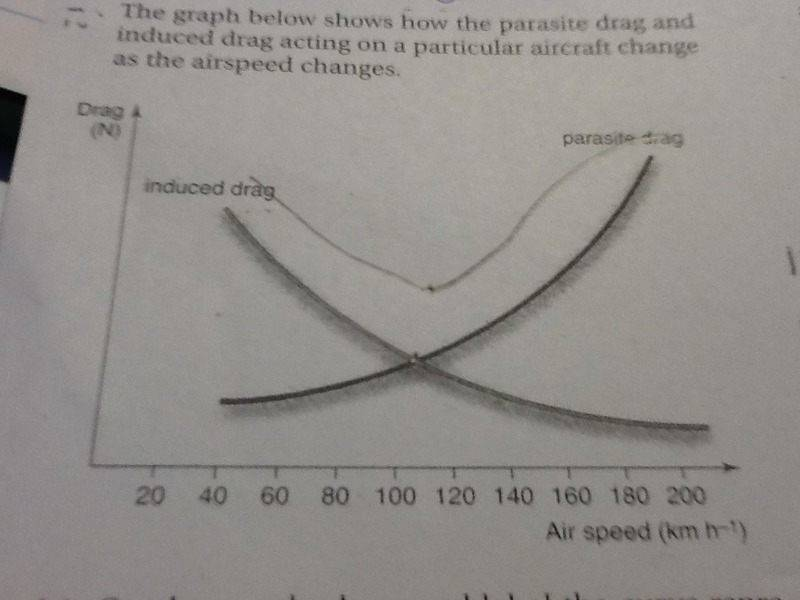# Physics-flight help

marinepyre
I need help with understanding bernoulli's principle, how an aerofoil develops lift i have horrible knowledge and understanding on how it works
And how the aerofoil produces lift in regards to bernoulli's principle.
Diagrams would help alot
Thanks

## Answers and Replies

Homework Helper
The simple explanation is that using a wing as a frame of reference, the wing diverts the relative air flow downwards. This corresponds to the wing exerting a downwards force onto the air, and the air exerting an upwards force on the wing. The exertion of a downwards force on the air corresponds to a lower pressure above the wing and/or a higher pressure below the wing. Air accelerates from higher pressure areas towards lower pressure areas, and as the air accelerates, Bernoulli equations notes a relationship between the decreasing pressure and increasing speed, assuming no work is done, and ignoring issues related to turbulence and friction with the wing. A wing performs some work on the air (using the air as a frame of reference, a wing adds energy to the air), and the work related portion of lift violates Bernoulli.

Last edited:
marinepyre
How does the air going downwards make air go upwards i don't understand
Im sorry

marinepyre
I also have another problem in regards to finding the maximum lift to drag ratio
I have been given a graph of airspeed over drag graph where airspeed is x axis and drag is y axisI have to find out maximum lift to drag ratio at what airspeed and when it occurs

Homework Helper
How does the air going downwards make air go upwards i don't understand.
The air doesn't have a net upwards flow. The net acceleration is downwards and the net velocity aft of the trailing edge of a wing is downwards. There's a Newton third law pair of forces, the wing exerts a downwards force onto the air, and the air reacts with an upwards force on the wing and also reacts by accelerating downwards. Assuming this is level flight, gravity keeps the aircraft flying level instead of accelerating upwards.

I also have another problem in regards to finding the maximum lift to drag ratio ... I have been given a graph of airspeed over drag graph where airspeed is x axis and drag is y axis.
There's not sufficient information. The maximium lift to drag ratio occurs where the lines cross, but there's no scale on the drag axis, and there's no information about the weight of the aircraft to compare the drag force against, even if the drag axis had a scale.

marinepyre
Thanks